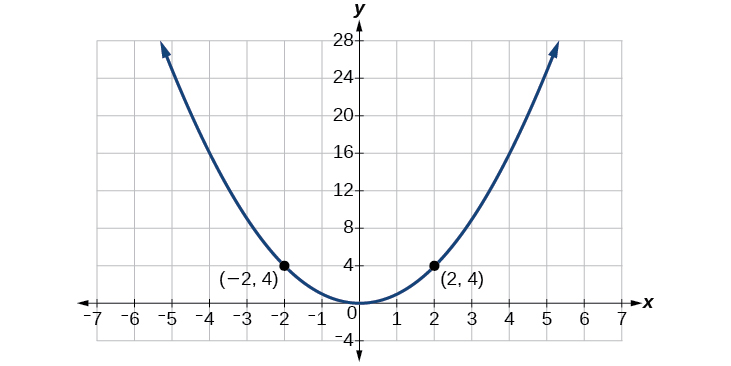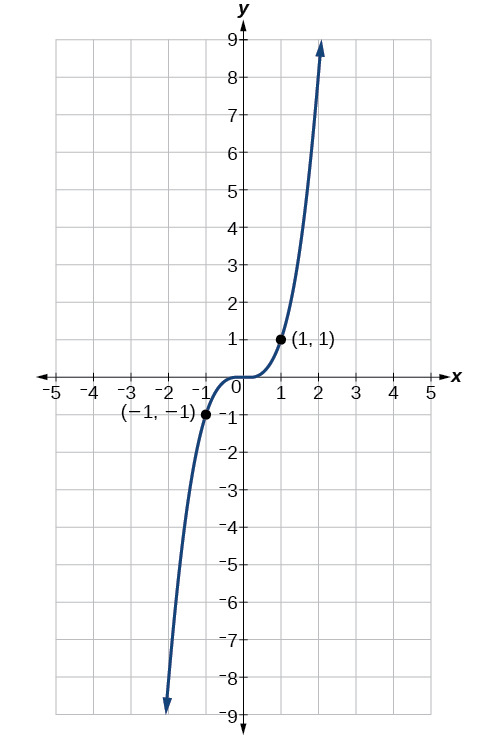# 7.4 The other trigonometric functions  (Page 3/14)

 Page 3 / 14

Use reference angles to find all six trigonometric functions of $\text{\hspace{0.17em}}-\frac{7\pi }{4}.$

$\begin{array}{l}\\ \mathrm{sin}\left(\frac{-7\pi }{4}\right)=\frac{\sqrt{2}}{2},\mathrm{cos}\left(\frac{-7\pi }{4}\right)=\frac{\sqrt{2}}{2},\mathrm{tan}\left(\frac{-7\pi }{4}\right)=1,\\ \mathrm{sec}\left(\frac{-7\pi }{4}\right)=\sqrt{2},\mathrm{csc}\left(\frac{-7\pi }{4}\right)=\sqrt{2},\mathrm{cot}\left(\frac{-7\pi }{4}\right)=1\end{array}$

## Using even and odd trigonometric functions

To be able to use our six trigonometric functions freely with both positive and negative angle inputs, we should examine how each function treats a negative input. As it turns out, there is an important difference among the functions in this regard.

Consider the function $\text{\hspace{0.17em}}f\left(x\right)={x}^{2},$ shown in [link] . The graph of the function is symmetrical about the y -axis. All along the curve, any two points with opposite x -values have the same function value. This matches the result of calculation: $\text{\hspace{0.17em}}{\left(4\right)}^{2}={\left(-4\right)}^{2},{\left(-5\right)}^{2}={\left(5\right)}^{2},$ and so on. So $\text{\hspace{0.17em}}f\left(x\right)={x}^{2}\text{\hspace{0.17em}}$ is an even function, a function such that two inputs that are opposites have the same output. That means $\text{\hspace{0.17em}}f\left(-x\right)=f\left(x\right).$The function   f ( x ) = x 2   is an even function.

Now consider the function $\text{\hspace{0.17em}}f\left(x\right)={x}^{3},$ shown in [link] . The graph is not symmetrical about the y -axis. All along the graph, any two points with opposite x -values also have opposite y -values. So $\text{\hspace{0.17em}}f\left(x\right)={x}^{3}\text{\hspace{0.17em}}$ is an odd function, one such that two inputs that are opposites have outputs that are also opposites. That means $\text{\hspace{0.17em}}f\left(-x\right)=-f\left(x\right).$The function   f ( x ) = x 3   is an odd function.

We can test whether a trigonometric function is even or odd by drawing a unit circle with a positive and a negative angle, as in [link] . The sine of the positive angle is $\text{\hspace{0.17em}}y.\text{\hspace{0.17em}}$ The sine of the negative angle is $\text{\hspace{0.17em}}-y.\text{\hspace{0.17em}}$ The sine function, then, is an odd function. We can test each of the six trigonometric functions in this fashion. The results are shown in [link] .

## Even and odd trigonometric functions

An even function is one in which $\text{\hspace{0.17em}}f\left(-x\right)=f\left(x\right).$

An odd function is one in which $\text{\hspace{0.17em}}f\left(-x\right)=-f\left(x\right).$

Cosine and secant are even:

Sine, tangent, cosecant, and cotangent are odd:

## Using even and odd properties of trigonometric functions

If the secant of angle $\text{\hspace{0.17em}}t\text{\hspace{0.17em}}$ is 2, what is the secant of $\text{\hspace{0.17em}}-t?$

Secant is an even function. The secant of an angle is the same as the secant of its opposite. So if the secant of angle $\text{\hspace{0.17em}}t\text{\hspace{0.17em}}$ is 2, the secant of $\text{\hspace{0.17em}}-t\text{\hspace{0.17em}}$ is also 2.

If the cotangent of angle $\text{\hspace{0.17em}}t\text{\hspace{0.17em}}$ is $\text{\hspace{0.17em}}\sqrt{3},$ what is the cotangent of $\text{\hspace{0.17em}}-t?$

$-\sqrt{3}$

## Recognizing and using fundamental identities

We have explored a number of properties of trigonometric functions. Now, we can take the relationships a step further, and derive some fundamental identities. Identities are statements that are true for all values of the input on which they are defined. Usually, identities can be derived from definitions and relationships we already know. For example, the Pythagorean Identity    we learned earlier was derived from the Pythagorean Theorem and the definitions of sine and cosine.

## Fundamental identities

We can derive some useful identities    from the six trigonometric functions. The other four trigonometric functions can be related back to the sine and cosine functions using these basic relationships:

$\mathrm{tan}\text{\hspace{0.17em}}t=\frac{\mathrm{sin}\text{\hspace{0.17em}}t}{\mathrm{cos}\text{\hspace{0.17em}}t}$
$\mathrm{sec}\text{\hspace{0.17em}}t=\frac{1}{\mathrm{cos}\text{\hspace{0.17em}}t}$
$\mathrm{csc}\text{\hspace{0.17em}}t=\frac{1}{\mathrm{sin}\text{\hspace{0.17em}}t}$
$\text{cot}\text{\hspace{0.17em}}t=\frac{1}{\text{tan}\text{\hspace{0.17em}}t}=\frac{\text{cos}\text{\hspace{0.17em}}t}{\text{sin}\text{\hspace{0.17em}}t}$

what are you up to?
nothing up todat yet
Miranda
hi
jai
hello
jai
Miranda Drice
jai
aap konsi country se ho
jai
which language is that
Miranda
I am living in india
jai
good
Miranda
what is the formula for calculating algebraic
I think the formula for calculating algebraic is the statement of the equality of two expression stimulate by a set of addition, multiplication, soustraction, division, raising to a power and extraction of Root. U believe by having those in the equation you will be in measure to calculate it
Miranda
state and prove Cayley hamilton therom
hello
Propessor
hi
Miranda
the Cayley hamilton Theorem state if A is a square matrix and if f(x) is its characterics polynomial then f(x)=0 in another ways evey square matrix is a root of its chatacteristics polynomial.
Miranda
hi
jai
hi Miranda
jai
thanks
Propessor
welcome
jai
What is algebra
algebra is a branch of the mathematics to calculate expressions follow.
Miranda
Miranda Drice would you mind teaching me mathematics? I think you are really good at math. I'm not good at it. In fact I hate it. 😅😅😅
Jeffrey
lolll who told you I'm good at it
Miranda
something seems to wispher me to my ear that u are good at it. lol
Jeffrey
lolllll if you say so
Miranda
but seriously, Im really bad at math. And I hate it. But you see, I downloaded this app two months ago hoping to master it.
Jeffrey
which grade are you in though
Miranda
oh woww I understand
Miranda
Jeffrey
Jeffrey
Miranda
how come you finished in college and you don't like math though
Miranda
gotta practice, holmie
Steve
if you never use it you won't be able to appreciate it
Steve
I don't know why. But Im trying to like it.
Jeffrey
yes steve. you're right
Jeffrey
so you better
Miranda
what is the solution of the given equation?
which equation
Miranda
I dont know. lol
Jeffrey
Miranda
Jeffrey
answer and questions in exercise 11.2 sums
how do u calculate inequality of irrational number?
Alaba
give me an example
Chris
and I will walk you through it
Chris
cos (-z)= cos z .
cos(- z)=cos z
Mustafa
what is a algebra
(x+x)3=?
6x
Obed
what is the identity of 1-cos²5x equal to?
__john __05
Kishu
Hi
Abdel
hi
Ye
hi
Nokwanda
C'est comment
Abdel
Hi
Amanda
hello
SORIE
Hiiii
Chinni
hello
Ranjay
hi
ANSHU
hiiii
Chinni
h r u friends
Chinni
yes
Hassan
so is their any Genius in mathematics here let chat guys and get to know each other's
SORIE
I speak French
Abdel
okay no problem since we gather here and get to know each other
SORIE
hi im stupid at math and just wanna join here
Yaona
lol nahhh none of us here are stupid it's just that we have Fast, Medium, and slow learner bro but we all going to work things out together
SORIE
it's 12
what is the function of sine with respect of cosine , graphically
tangent bruh
Steve
cosx.cos2x.cos4x.cos8x
sinx sin2x is linearly dependent
what is a reciprocal
The reciprocal of a number is 1 divided by a number. eg the reciprocal of 10 is 1/10 which is 0.1
Shemmy
Reciprocal is a pair of numbers that, when multiplied together, equal to 1. Example; the reciprocal of 3 is ⅓, because 3 multiplied by ⅓ is equal to 1
Jeza
each term in a sequence below is five times the previous term what is the eighth term in the sequence
I don't understand how radicals works pls
How look for the general solution of a trig functionByByBy Yasser IbrahimBy Madison ChristianBy OpenStaxBy OpenStaxBy Saylor FoundationBy Anh DaoBy Anindyo MukhopadhyayBy OpenStaxBy Jessica CollettBy Nick Swain